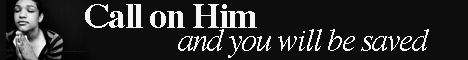Note:  Do not rely on this information. It is very old.

# Triangleof Velocities

Triangle of Velocities is merely a modification of the parallelogram of velocities. Thus if the line O A represents a velocity, V1, in magnitude and direction, and if from the end A of this line another line, A B, be drawn to similarly represent a second velocity, V2, then the line O B of the triangle O A B will represent the resultant of V1 and V2. [PARALLELOGRAM OF VELOCITIES.]